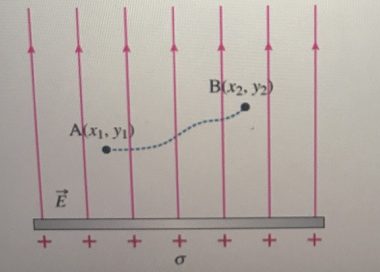# Problem: Let A  = (x1, y1) and B = (x2, y2) be two points near and on the same side of a charged sheet with surface charge density+σ. The electric field E due to such a charges sheet has magnitude E = σ/2e0 everywhere, and the field points away from the sheet, as shown in the diagram.Part A. What is the potential difference VAB = VA – VB between points A and B?Part B. If the potential at y = ±∞ is taken to be zero, what is the value of the potential at a point VA at some positive distance y1 from the surface of the sheet?Choices area. ∞b. -∞c. 0d. -E•y1

###### FREE Expert Solution

The potential difference between points A and B can be expressed as:

$\overline{){{\mathbf{V}}}_{\mathbf{A}\mathbf{B}}{\mathbf{=}}{\mathbf{-}}{{\mathbf{\int }}}_{{\mathbf{B}}}^{{\mathbf{A}}}{\mathbf{E}}{\mathbf{·}}{\mathbf{d}}{\mathbf{l}}}$

96% (256 ratings)###### Problem Details

Let A  = (x1, y1) and B = (x2, y2) be two points near and on the same side of a charged sheet with surface charge density
+σ. The electric field E due to such a charges sheet has magnitude E = σ/2e0 everywhere, and the field points away from the sheet, as shown in the diagram.Part A. What is the potential difference VAB = VA – VB between points A and B?

Part B. If the potential at y = ±∞ is taken to be zero, what is the value of the potential at a point VA at some positive distance y1 from the surface of the sheet?

Choices are
a. ∞

b. -∞

c. 0

d. -E•y1

Frequently Asked Questions

What scientific concept do you need to know in order to solve this problem?

Our tutors have indicated that to solve this problem you will need to apply the Potential Difference with Calculus concept. You can view video lessons to learn Potential Difference with Calculus. Or if you need more Potential Difference with Calculus practice, you can also practice Potential Difference with Calculus practice problems.